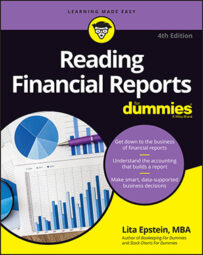##### Reading Financial Reports For DummiesYou can measure a company's cash position to meet long-term debt needs by using financial reports to determine the cash debt coverage ratio. Long-term liabilities are debts that a company must pay beyond that 12-month period. If you see signs that a firm may have difficulties meeting long-term debt, that, is a major cause for concern.

Although you may find that a company generates enough cash to meet its current liabilities, if long-term debt levels are too high, the company will eventually run into trouble paying off its debt and meeting its interest obligations. The formula for the cash debt coverage ratio is a two-step process:

1. Find the average total liabilities.

(Current year total liabilities + Previous year total liabilities) ÷2 = Average total liabilities

2. Find the cash debt coverage ratio.

Cash provided by operating activities ÷Average total liabilities = Cash debt coverage ratio

You can find the current- and previous-year total liabilities on the balance sheet. You can find cash provided by operating activities on the statement of cash flows.

## Mattel

Using the cash provided by operating activities from Mattel's 2012 cash flow statement and the average of its total liabilities from its 2012 and 2011 balance sheets, this is how to calculate the cash debt coverage ratio. Using the two-step process, you first calculate the average total liabilities; then you use that number to calculate the ratio:

1. Calculate average total liabilities.

\$3,459,741,000 (2012 total liabilities) + \$2,818,008,000 (2011 total liabilities) ÷2 = \$3,260,388,000 (Average total liabilities)

2. Find the cash debt coverage ratio.

\$1,275,650,000 (2012 cash provided by operating activities) ÷\$3,260,388,000 (Average total liabilities) = 0.39 (Cash debt coverage ratio)

To judge whether a company's cash provided by activities is improving, you calculate the ratio for both the current reporting year and the previous reporting year:

\$664,693,000 (2012 cash provided by operating activities) ÷ \$3,260,388,000 (Average total liabilities) = 0.20 (Cash debt coverage ratio)

This ratio serves as evidence that Mattel's cash position improved from the end of 2011 to the end of 2012. Its cash position worsened because less cash came into the company from inventories and more went out in accounts payable. Mattel's total debt increased between 2011 and 2012 by about \$398.7 million, according to its balance sheet.

## Hasbro

To show you how to calculate the cash debt coverage ratio, you use the cash provided by operating activities from Hasbro's 2012 cash flow statement and the average of its total liabilities from its 2012 and 2011 balance sheets:

1. Calculate average total liabilities.

\$2,818,008,000 (2012 total liabilities) + \$2,713,259,000 (2011 total liabilities) ÷2 = \$2,765,634,000 (Average total liabilities)

2. Calculate the cash debt coverage ratio for the current reporting year.

\$534,796,000 (2012 cash provided by operating activities) ÷\$2,765,634,000 (Average total liabilities) = 0.19 (Cash debt coverage ratio)

Calculate the ratio for the previous year as well:

\$396,069,000 (2011 cash provided by operating activities) ÷ \$2,765,634,000 (Average total liabilities) = 0.14 (Cash debt coverage ratio)

Taking total liabilities into consideration, Hasbro ended 2012 in a stronger cash position than in 2011, but Mattel's cash improvement was much greater. Much of that improvement, though, came from the delay in payments, as discussed earlier.

## What do the numbers mean?

The cash debt coverage ratio looks at a company's ability to pay its long-term debt obligations. As you can see, when long-term debt is taken into consideration, Mattel's cash position was still better than Hasbro's, but not as strong as when looking only at current liabilities. Both Mattel and Hasbro carry a larger part of their debt as long-term debt.

So calculating only one ratio — current cash debt coverage ratio or cash debt coverage ratio — doesn't give you the full picture of a company's financial health. You need to look at both ratios to be certain that the company is generating enough cash to cover both its short-term and long-term debt.

As with the current cash debt coverage ratio, if you find a negative cash from operations number, be sure to look for an explanation in the notes to the financial statements or in the management's discussion and analysis to find out why the cash flow from operations is negative. If you don't find it there, call the company's investor relations office to get the answers to your questions.